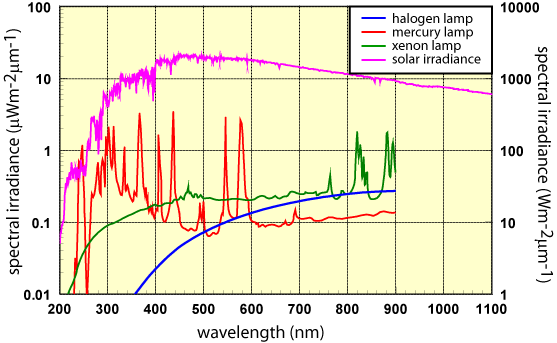The spectral irradiance as a function of photon wavelength (or energy), denoted by F, is the most common way of characterising a light source. It gives the power density at a particular wavelength. The units of spectral irradiance are in Wm-2µm-1. The Wm-2 term is the power density at the wavelength λ(µm). Therefore, the m-2 refers to the surface area of the light emitter and the µm-1 refers to the wavelength of interest.

In the analysis of solar cells, the photon flux is often needed as well as the spectral irradiance. The spectral irradiance can be determined from the photon flux by converting the photon flux at a given wavelength to W/m2 as shown in the section on Photon Flux. The result is then divided by the given wavelength, as shown in the equation below.

## Photon Flux

where in SI units:
F(λ) is the spectral irradiance in Wm-2μm-1;
Φ is the photon flux in # photons m-2sec-1;
E and λ are the energy and wavelength of the photon in joules and meters respectively; and

The spectral irradiance is more commonly expressed in terms of wavelength so that:

## Photon Flux : Wavelength

where:
F(λ) is the spectral irradiance in Wm-2µm-1;
Φ is the photon flux in # photons m-2sec-1;
E and λ are the energy and wavelength of the photon in eV and µm respectively; and
q is a constant of 1.6 · 10-19The spectral irradiance of artificial light sources (left axis) compared to the spectral irradiance from the sun (right axis).